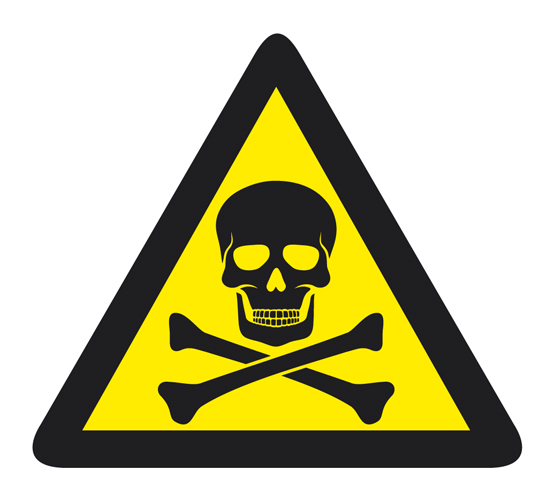• ## Accessibility

Open the accessibility toolbar to change fonts and contrast, access a dictionary, use a ruler and more

Who invented the round table?

Sir Cumference!Circumference of a circle just means the distance around the circle.  Sometimes it is also called the perimeter of the circle.

There are a few other things you need to know.

DiameterThe diameter is the line that cuts through the centre of the circle.The radius comes from the centre of the circle to the edge and is half the diameter.

PiNo, not that pie this one.You will need to learn the value for this..sorry. Did you know pi is infinite, some people can quote it to hundreds of decimal places?

Lucky for you that you only need to use it to 3 decimal places which is 3.142

Finally, you need the formula for finding the circumference of a circle

Circumference of a circle  = Pi x diameter  or C = pi x d

A great way to remember it is Cherry Pies are deliciousBuilding a toolbox is useful.  Builders have them so do mathematicians as we are building our knowledge.

Our toolbox contains definitions for Circumference, Perimeter, Diameter, Radius, Pi and the Formula. Looking good.

Let's get startedCalculate the circumference of this circle.

Pi x diameter

3.142 x 14 = 43.988 cm  (42.99cm to 2 decimal places)

You will be asked to round to decimal places or significant figures.

Warning!You may get a question that gives you the radius.

Remember the radius is only half of the diameter so double it first.

Calculate the circumference of a circle with a radius of 3 cm.

3 + 3 = 6 cm diameter

Now apply the formula Pi x diameter. 3.142 x 6 = 18.852  (18.9 to 3 significant figures)

Use the π button on your calculator to work out the following answers if you don't have a calculator to hand you can use a scientific calculator online.

10 questions